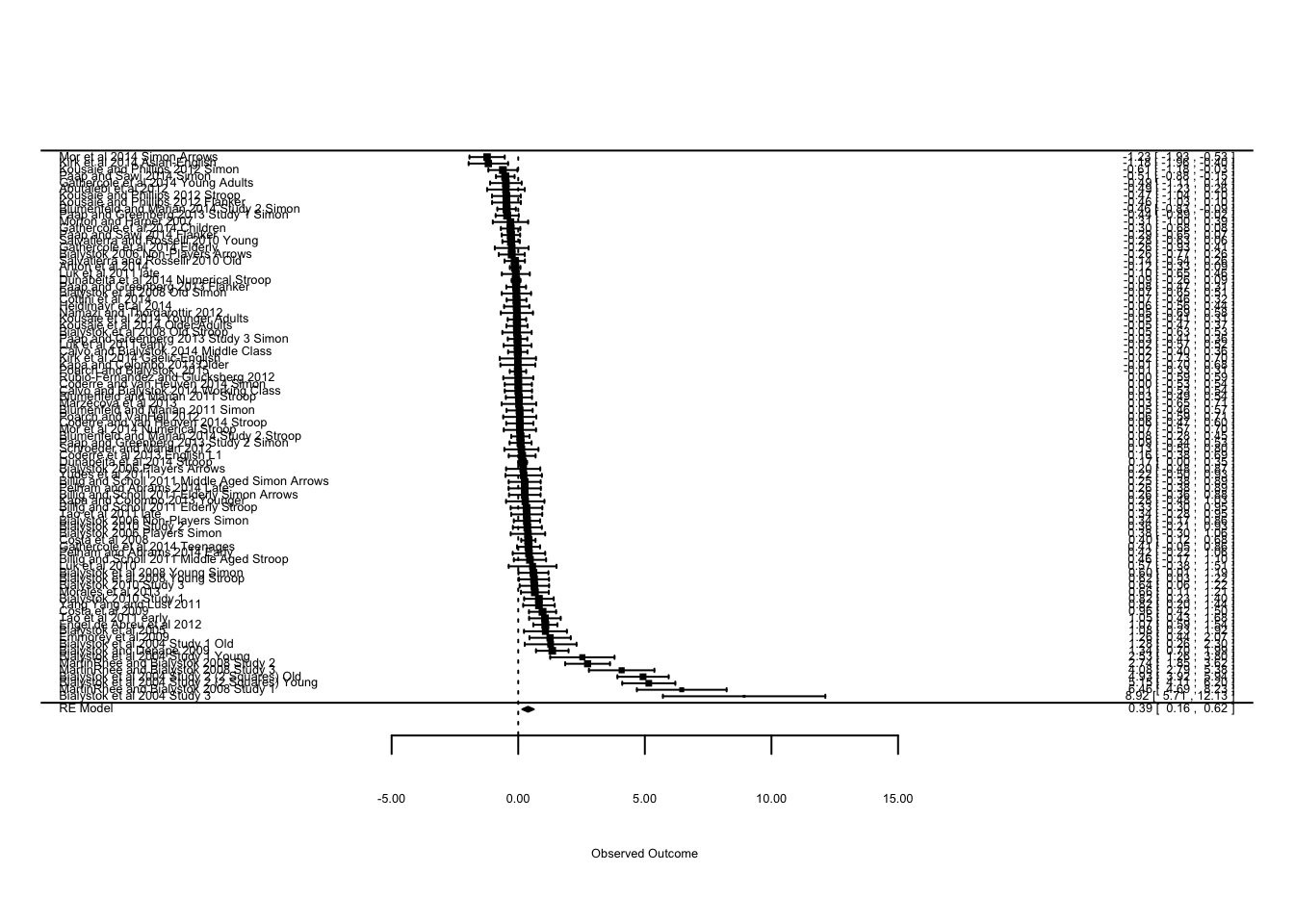This script runs the analyses of the interference control tasks assuming a correlation between congruent and incongruent trials of 0. This is a form of sensitivity analysis to determine how sensitivie my inferences are to different assumed correlations between these trial types.
The script is almost identical to the primary script, except that instead of randomly simulating correlation coefficients, I create a vector of zeroes.

We need metaSEM, metafor and probably ggplot

``````library(metaSEM)
library(metafor)
library(ggplot2)``````

Next we need to generate correlation coefficients and insert them into the dataframe for studies that don’t have an author-provided correlation

``````full_set2_im <- read.csv("~/Dropbox/Working!/Studies For Meta-Analysis/Analysis/Main Meta Analysis/full_set2_im.csv")
corrs <- rnorm(86, 0.0, 0.047)

for(i in 1:86){
if(is.na(full_set2_im\$Bi_Corr_Imputed[i])) {full_set2_im\$Bi_Corr_Imputed[i] = corrs[i]}
if(is.na(full_set2_im\$Mono_Corr_Imputed[i])) {full_set2_im\$Mono_Corr_Imputed[i] = corrs[i]}
}  ``````
``````### Preparing the Dataset
##Estimating the overall RTs and interference costs

for (i in 1:86){
if(is.na(full_set2_im\$Bi_Overall[i]))
full_set2_im\$Bi_Overall[i] = (full_set2_im\$Bi_Congruent[i] + full_set2_im\$Bi_Incongruent[i])/2
}
for (i in 1:86){
if(is.na(full_set2_im\$Mon_Overall[i]))
full_set2_im\$Mon_Overall[i] = (full_set2_im\$Mon_Congruent[i] + full_set2_im\$Mon_Incongruent[i])/2
}
#Interference Cost
for (i in 1:86){
if(is.na(full_set2_im\$Bi_Int[i]))
full_set2_im\$Bi_Int[i] = full_set2_im\$Bi_Incongruent[i] - full_set2_im\$Bi_Congruent[i]
}
for (i in 1:86){
if(is.na(full_set2_im\$Mon_Int[i]))
full_set2_im\$Mon_Int[i] = full_set2_im\$Mon_Incongruent[i] - full_set2_im\$Mon_Congruent[i]
}

##Estimating SDs of costs.
#Overall RT
for (i in 1:86){
if(is.na(full_set2_im\$Bi_Overall_SD[i]))
full_set2_im\$Bi_Overall_SD[i] = sqrt((full_set2_im\$Bi_Congruent_SD[i]^2 + full_set2_im\$Bi_Incongruent_SD[i]^2 + 2*full_set2_im\$Bi_Corr_Imputed[i]*full_set2_im\$Bi_Congruent_SD[i]*full_set2_im\$Bi_Incongruent_SD[i]))/2
}
for(i in 1:86){
if(is.na(full_set2_im\$Mon_Overall_SD[i]))
full_set2_im\$Mon_Overall_SD[i] = sqrt((full_set2_im\$Mon_Congruent_SD[i]^2 + full_set2_im\$Mon_Incongruent_SD[i]^2 + 2*full_set2_im\$Mono_Corr_Imputed[i]*full_set2_im\$Mon_Congruent_SD[i]*full_set2_im\$Mon_Incongruent_SD[i]))/2
}
#Interference Cost
for (i in 1:86){
if(is.na(full_set2_im\$Bi_Int_SD[i]))
full_set2_im\$Bi_Int_SD[i] = sqrt((full_set2_im\$Bi_Congruent_SD[i]^2 + full_set2_im\$Bi_Incongruent_SD[i]^2 - 2*full_set2_im\$Bi_Corr_Imputed[i]*full_set2_im\$Bi_Congruent_SD[i]*full_set2_im\$Bi_Incongruent_SD[i]))
}
for (i in 1:86){
if(is.na(full_set2_im\$Mon_Int_SD[i]))
full_set2_im\$Mon_Int_SD[i] = sqrt((full_set2_im\$Mon_Congruent_SD[i]^2 + full_set2_im\$Mon_Incongruent_SD[i]^2 - 2*full_set2_im\$Mono_Corr_Imputed[i]*full_set2_im\$Mon_Congruent_SD[i]*full_set2_im\$Mon_Incongruent_SD[i]))
}

##Calculating_Effect_Size
## Monitoring
full_set2_im\$monitoring_diff <- with(full_set2_im, Mon_Overall - Bi_Overall)
full_set2_im\$monitoring_sd <- with(full_set2_im, sqrt((((N_B - 1)*Bi_Overall_SD^2) + ((N_M - 1)*Mon_Overall_SD^2))/(N_B + N_M)))
full_set2_im\$monitoring <-with(full_set2_im,monitoring_diff/monitoring_sd)
full_set2_im\$monitoring_var <-with(full_set2_im, (((N_B+N_M)/(N_B*N_M)) + ((monitoring^2)/(2*(N_B+N_M-2))))*((N_B+N_M)/(N_B+N_M-2)))
## Interference Costs
full_set2_im\$interference_diff <- with(full_set2_im, Mon_Int - Bi_Int)
full_set2_im\$interference_sd <- with(full_set2_im, sqrt((((N_B - 1)*Bi_Int_SD^2) + ((N_M - 1)*Mon_Int_SD^2))/(N_B + N_M)))
full_set2_im\$interference_cost <- full_set2_im\$interference_diff/full_set2_im\$interference_sd
full_set2_im\$interference_cost_var <-with(full_set2_im, (((N_B+N_M)/(N_B*N_M)) + ((interference_cost^2)/(2*(N_B+N_M-2))))*((N_B+N_M)/(N_B+N_M-2)))
##Creating age factor
full_set2_im\$Age_Group <- rep(0, 86)
for(i in 1:86){
if(full_set2_im\$Age[i] < 13) {full_set2_im\$Age_Group[i] = 1}
else if(full_set2_im\$Age[i] > 18 & full_set2_im\$Age[i] <40) {full_set2_im\$Age_Group[i] = 2}
else if(full_set2_im\$Age[i] > 40 & full_set2_im\$Age[i] <60) {full_set2_im\$Age_Group[i] = 3}
else if (full_set2_im\$Age[i] >= 60) {full_set2_im\$Age_Group[i] =4}
}

full_set2_im <- subset(full_set2_im, Task != "Simon_2")``````

Before running the analysis, we’ll make forest plots for the global RT and interference costs.

``forest(rma(yi=monitoring, vi=monitoring_var, data=full_set2_im), order="obs", slab=full_set2_im\$Study, cex=.4);````summary(rma(yi=monitoring, vi=monitoring_var, data=full_set2_im))``
``````##
## Random-Effects Model (k = 84; tau^2 estimator: REML)
##
##    logLik   deviance        AIC        BIC       AICc
## -137.6321   275.2643   279.2643   284.1020   279.4143
##
## tau^2 (estimated amount of total heterogeneity): 1.0261 (SE = 0.1756)
## tau (square root of estimated tau^2 value):      1.0130
## I^2 (total heterogeneity / total variability):   94.49%
## H^2 (total variability / sampling variability):  18.16
##
## Test for Heterogeneity:
## Q(df = 83) = 548.6690, p-val < .0001
##
## Model Results:
##
## estimate       se     zval     pval    ci.lb    ci.ub
##   0.3874   0.1164   3.3292   0.0009   0.1593   0.6155      ***
##
## ---
## Signif. codes:  0 '***' 0.001 '**' 0.01 '*' 0.05 '.' 0.1 ' ' 1``````
``forest(rma(yi=interference_cost, vi=interference_cost_var, data=full_set2_im), order="obs", slab=full_set2_im\$Study, cex=.4)``